Select Page

# CBSE MCQ in English Answers for Maths 12 Science Application of Derivatives

CBSE MCQ in English Answers for Maths 12 Science Application of Derivatives to enable students to get Answers in a narrative video format for the specific question.

Expert Teacher provides CBSE MCQ Answers for Maths 12 Science Application of Derivatives through Video Answers in English language. This video solution will be useful for students to understand how to write an answer in exam in order to score more marks. This teacher uses a narrative style for a question from Application of Derivatives not only to explain the proper method of answering question, but deriving right answer too.

Please find the question below and view the Answer in a narrative video format.

Question:

## Similar Questions from CBSE, 12th Science, Maths, Application of Derivatives

Question 1 : The line y = mx + 1 is a tangent to the curve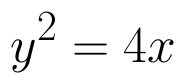if the value of m is: (View Answer Video)

Question 2 : Find two positive numbers x  and y such that x+y=60 and is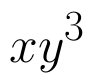maximum. (View Answer Video)

Question 3 : The point on the curve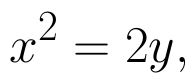which is nearest to the point (0, 5) is :

Question 4 : A balloon, which always remains spherical has a variable radius. Find the rate at which its volume is increasing with the radius when the later is 10 cm.  (View Answer Video)

Question 5 : Sand is pouring from a pipe at the rate of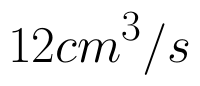. The falling sand forms a cone on the ground in such a way that the height of the cone is always one-sixth of the radius of the base. How fast is the height of the sand cone increasing, when the height is 4 cm? (View Answer Video)

### Continuity and Differentiability

Question 1 : Differentiate the function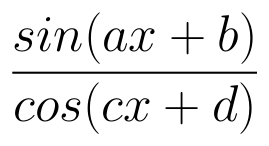with respect to x. (View Answer Video)

Question 2 : Differentiate w.r.t.x the function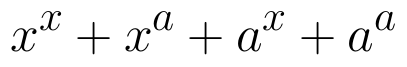, for some fixed a > 0 and x > 0. (View Answer Video)

Question 3 : Differentiate the function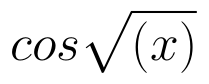with respect to x. (View Answer Video)

Question 4 : Findfor the function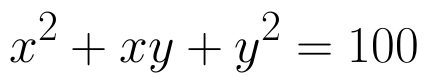. (View Answer Video)

Question 5 : Differentiate the function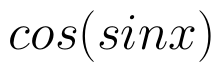with respect to x. (View Answer Video)

### Determinant

Question 1 : Find the equation of the line joining (1, 2) and (3, 6) using determinants. (View Answer Video)

Question 2 : Evaluate the determinant: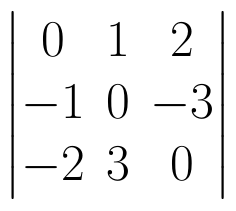. (View Answer Video)

Question 4 : If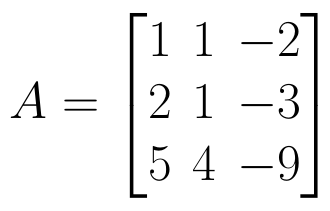find |A|. (View Answer Video)

Question 5 :  Evaluate the determinants: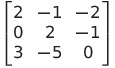. (View Answer Video)

### Application of Integrals

Question 1 :

Find the area of the given curves and given lines: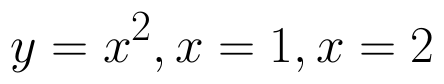and x-axis

Question 3 : Find the area enclosed by the parabola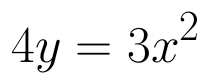and the line 2y = 3x + 12.   (View Answer Video)
Question 4 : Find the area bounded by curves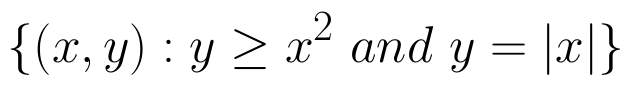. (View Answer Video)
Question 5 : Find the area of the region bounded by the curves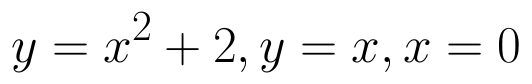and x = 3. (View Answer Video)